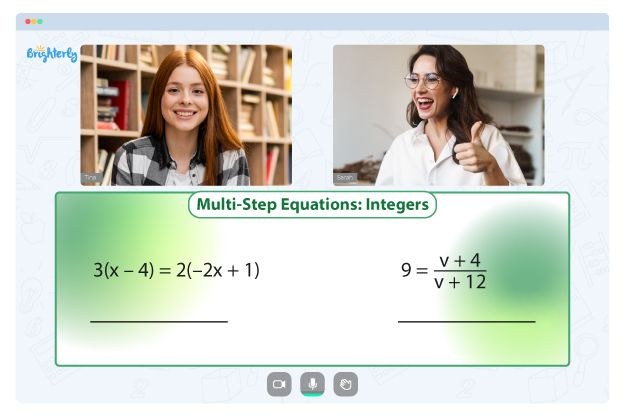# One Step Equations Worksheets

One step equations are mathematical equations that can be solved using a single operation, such as addition, subtraction, multiplication, or division. These equations are often used in elementary and middle school mathematics to teach students the fundamentals of algebra.

To solve a one step equation, students typically begin by identifying the operation performed on the variable and then apply the inverse operation to isolate the variable on one side of the equation. For example, if the equation is 2x + 3 = 9, students would first subtract 3 from both sides to get 2x = 6 and then divide both sides by 2 to get x = 3.

One step equation worksheets allow students to practice solving a variety of one step equations and to gain a deeper understanding of the underlying principles and methods used in algebra.

## Benefits Of Worksheets One Step Equations

Here are some of the benefits of working with one-step equations worksheets:

Math for Kids

Is Your Child Struggling With Math?
1:1 Online Math Tutoring### They Help Students Reinforce Their Understanding Of One Step Equations:

One step algebraic equations worksheets are essential for students learning algebraic concepts as they provide a structured way to develop and practice the skills necessary for success in more advanced mathematics. In addition, by engaging in regular practice through these worksheets, students can build a strong foundation in one step equations, which can help them tackle more complex algebraic concepts with greater ease and confidence.

### They Provide A Visual Learning Approach

One of the valuable features of one step equations worksheets 7th grade is the inclusion of visual simulations that allow students to see mathematical concepts in action. Visual simulations are graphical representations demonstrating mathematical concepts engagingly and interactively for students.

Using visual simulations, students can see the relationships between numbers, equations, and graphs in a concrete and meaningful way. These simulations may include animations, diagrams, graphs, or other visual aids that demonstrate how mathematical concepts work in the real world.

### They Provide Clear And Concise Explanations Of How Students Can Work With The Equations

One of the critical features of one step algebra equations worksheets is the inclusion of clear and concise instructions, which are essential for helping students understand how to solve these equations step by step. The instructions in these worksheets are designed to be easily understood, even by students new to algebraic concepts.

Clear instructions often include a breakdown of each step needed to solve the equation, including the order in which the steps should be taken so that students understand the process and ensure they do not miss any essential steps.

### They Are Easily Customizable

Teachers can customize any worksheet one step equations to cater to students’ diverse classroom learning styles and levels.

Customization may involve varying the difficulty levels of the problems or changing the types of equations used in the worksheet. For example, some students may require simpler problems to build their confidence and foundational skills, while others may benefit from more complex problems that can challenge and engage them. Teachers may also modify the equations used in the worksheet to align with the specific needs of their students, such as incorporating real-world scenarios or examples relevant to their students’ interests.

### They Can Be Used For Assessment

One step equations worksheets enable teachers to evaluate their students’ understanding of the topic and identify areas where additional support may be required.

Regular use of these worksheets allows teachers to monitor students’ progress and gauge their mastery of one-step equations.

If you want to start using one step equations worksheets for your class, you can download free one step equations worksheets in printable PDF format from the internet for your kids to use. Additionally, you may decide to use colorful ones for them.

Problems with Equations?• Is your child finding it hard to grasp the equations of math?
• An online tutor could be of assistance.

Does your child struggle with understanding equations lessons? Try learning with an online tutor.